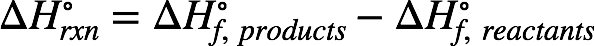# Problem: Hydrogen and methanol have both been proposed as alternatives to hydrocarbon fuels. Use standard enthalpies of formation to calculate the amount of heat released per kilogram of methanol fuel.

###### FREE Expert Solution

Recall: The enthalpy of reaction (ΔH˚rxn) can be calculated from the reactants' and products' enthalpy of formation (∆H˚f) using the equation:In the combustion, CH3OH(l) reacts with O2(g) to form CO2(g) and H2O(l). The chemical equation is:

CH3OH(l) + O2(g) → CO2(g) + H2O(l)

This equation is not yet balanced. To balance it, add a coefficient of 2 to H2O to balance the number of H. The equation currently looks like this:

CH3OH(l) + O2(g) → CO2(g) + 2 H2O(l)

The equation currently has 3 O in the reactant side and 4 O in the product side. We add a coefficient of 3/2 to O2 to balance the number of O. The balanced equation is:

CH3OH(l) + 3/2 O2(g) → CO2(g) + 2 H2O(l)###### Problem Details

Hydrogen and methanol have both been proposed as alternatives to hydrocarbon fuels. Use standard enthalpies of formation to calculate the amount of heat released per kilogram of methanol fuel.